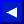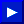### LEN

TYPE: Integer Function
Format: LEN (<string>)

Action: Returns the number of characters in the string expression. Non-printed characters and blanks are counted.

EXAMPLE of LEN Function:
```   CC\$ = "COMMODORE COMPUTER": PRINT LEN(CC\$)

18
```

### LET

TYPE: Statement
FORMAT: [LET] <variable> = <expression>

Action: The LET statement can be used to assign a value to a variable. But the word LET is optional and therefore most advanced programmers leave LET out because it's always understood and wastes valuable memory. The equal sign (=) alone is sufficient when assigning the value of an expression to a variable name.

EXAMPLES of LET Statement:
```   10 LET D= 12              (This is the same as D = 12)
20 LET E\$ = "ABC"
30 F\$ = "WORDS"
40 SUM\$= E\$ + F\$          (SUM\$ would equal ABCWORDS)
```This page has been created by Sami Rautiainen. Read the small print. Last updated November 07, 1998.